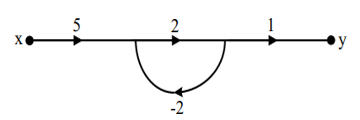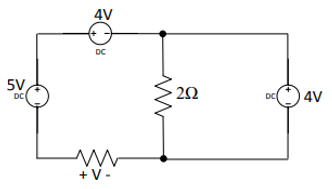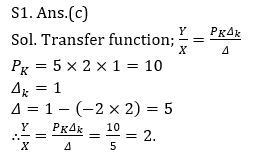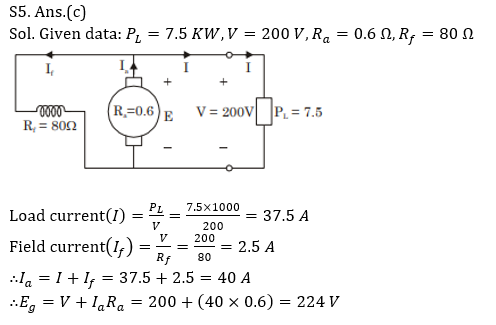Engineering Jobs   »   ELECTRICAL GATE QUIZ

# GATE’22 EE: Daily Practices Quiz 13-Aug-2021

Each question carries 2 marks
Negative marking: 2/3 mark

Total Questions: 05
Total marks: 10
Time: 12 min.

Q1. In the signal flow graph of the figure y/x equals(a) 3
(b)5/2
(c) 2
(d)None of the above

Q2. The Voltage V in Figure is equal to(a) 3 V
(b) -3 V
(c) 5 V
(d) None of these

Q3. Which one of the following statements regarding an effect of phase lead network is
NOT correct?
(a) The velocity constant is usually increased
(b) The slope of the magnitude curve is reduced at the gain crossover frequency, as a result relative stability improves
(c) Phase margin increased
(d) The bandwidth decreased

Q4. A transformer core is wound with a coil carrying an alternating current at a frequency
of 50 Hz. The hysteresis loop has an area of 60000 units when the axes are drawn in
units of 10^(–4) Wb m^(–2) and 10^2 Am^(–1). If the magnetization is uniform throughout the core
volume of 0.01 m^3, the power loss due to hysteresis will be
(a) 300 W
(b) 350 W
(c) 400 W
(d) 450 W

Q5. A DC shunt generator supplies a load of 7.5 kW at 200 V. If the armature resistance is 0.6 Ω and field resistance is 80 Ω, the induced emf will be
(a) 212 V
(b) 218 V
(c) 224 V
(d) 204 V

SOLUTIONSS2. Ans.(a)
Sol. Apply KVL
V + 5 – 4 = 4
V = 4 + 4 – 5
= 3V

S3. Ans.(d)
Sol. Effects of phase lead network:
1. Velocity constant K_v increases
2. The slope of the magnitude plot reduces at gain crossover frequency, as a result relative stability improves.
3. Phase margin increases
4. Response becomes faster
5. Increases the system bandwidth.

S4. Ans.(a)
Sol. Hysteresis loss = Area of Hysteresis loop × Frequency × Volume of core
= {(60000) (10^(–4) × 10^2) }(50) (0.01)
=6×50= 300 W.Sharing is caring!

Thank You, Your details have been submitted we will get back to you.